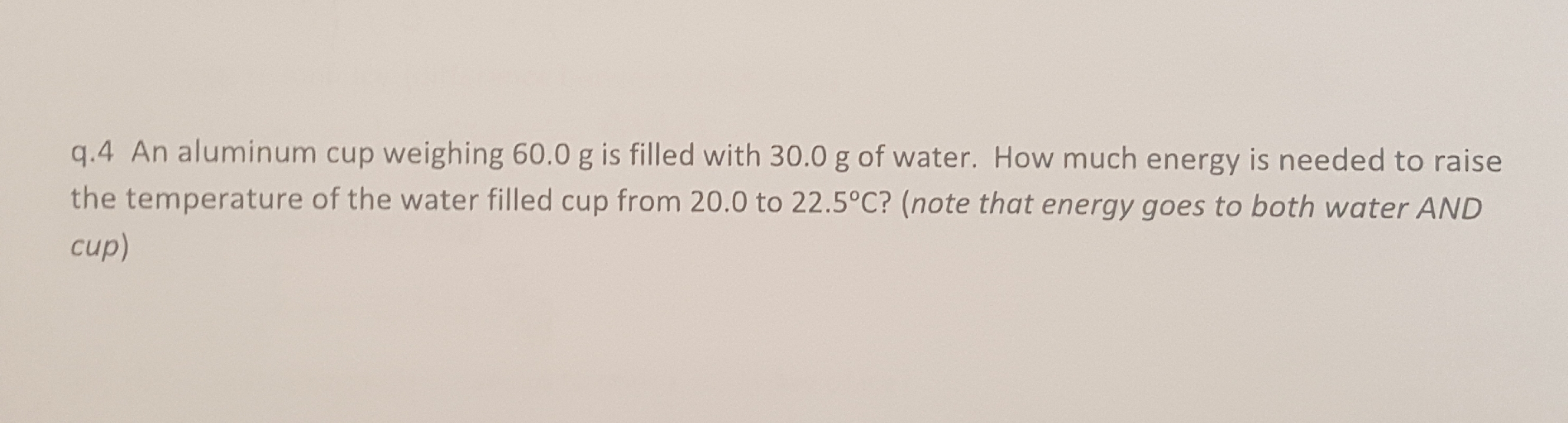# q.4 An aluminum cup weighing 60.0 g is filled with 30.0 g of water. How much energy is needed to raisethe temperature of the water filled cup from 20.0 to 22.5°C? (note that energy goes to both water ANDcup)

Question
1 viewshelp_outlineImage Transcriptioncloseq.4 An aluminum cup weighing 60.0 g is filled with 30.0 g of water. How much energy is needed to raise the temperature of the water filled cup from 20.0 to 22.5°C? (note that energy goes to both water AND cup) fullscreen
check_circle

Step 1

Specific heat is the heat absorbed or released by one gram of a substance during change of one degree centigrade or one Kelvin temperature .

Or

Heat capacity is the heat absorbed or released by a substance during change of one degree centigrade or one Kelvin temperature.

Step 2

Given:

Mass of water = 30.0 g.

Mass of aluminium cup = 60.0 g

Initial temperature of water = 20.0 0C.

Initial temperature of aluminium = 20.0 0C

Final temperature of both water and aluminium = 22.5 0C.

Formula used:

Q = ms ΔT

Where

Q = heat absorbed / released.

m = mass of substance.

s = specific heat of substance.

ΔT = temperature change.

Step 3

We know that:

Specific heat of aluminium = 0.900 J/gm 0C

Specific heat of water = 4.184 J/gm 0C

As given that both aluminium and water will absorb heat to raise the temperature.

Let us calculate the heat needed by each

Heat needed by water:

Q1 = mass ...

### Want to see the full answer?

See Solution

#### Want to see this answer and more?

Solutions are written by subject experts who are available 24/7. Questions are typically answered within 1 hour.*

See Solution
*Response times may vary by subject and question.
Tagged in

### Chemistry